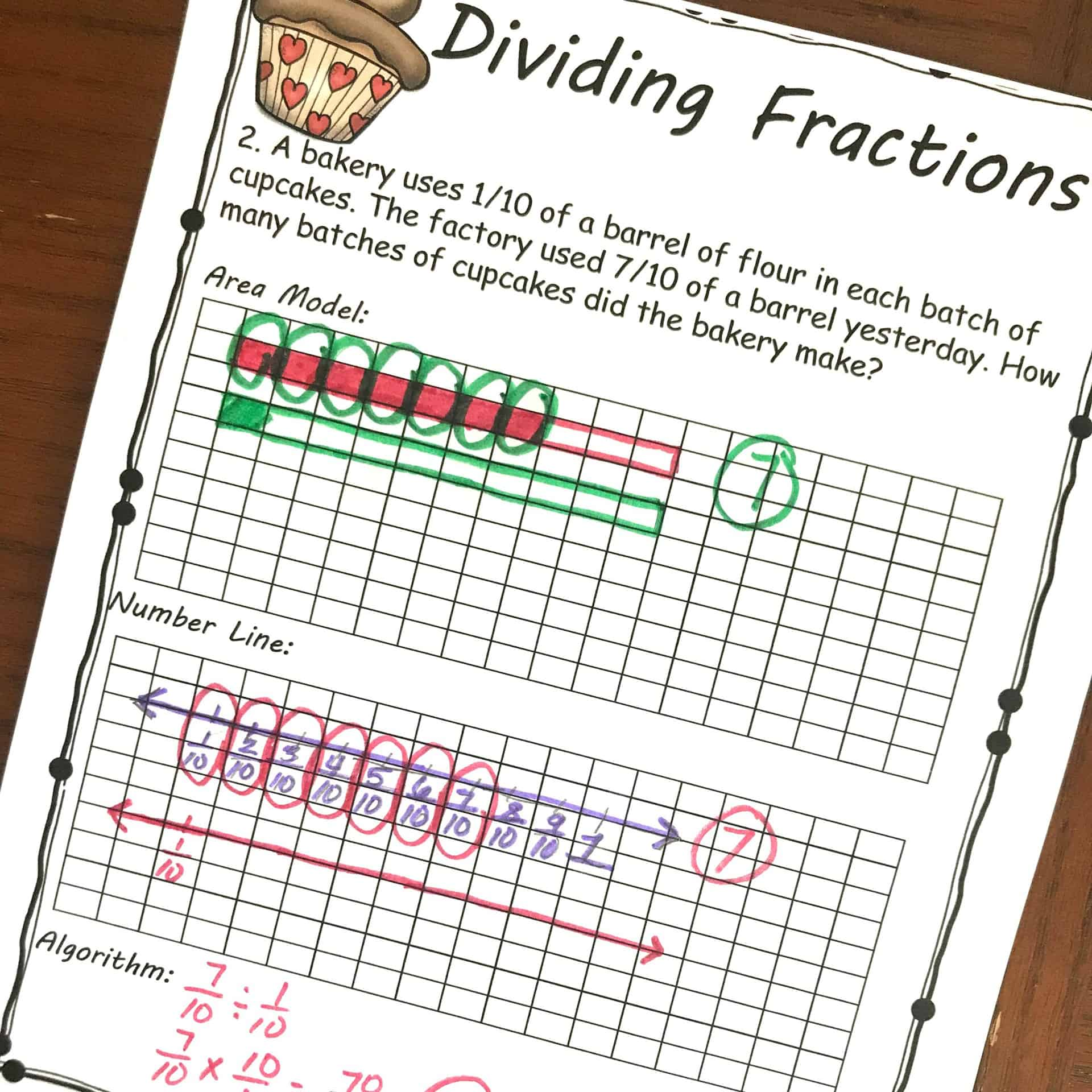# FREE Yummy Word Problems For Dividing Fractions by Fractions

These free word problems get children dividing fractions by fractions through area models, number lines, and algorithms.

## Get your freebie by clicking on the blue button at the VERY end of the post.

Dividing fractions!!

I have to admit, this is a hard one. Fractions can be complicated, division can be a challenge, and when you put the two together….WOW!

But today we are going to tackle this task!

So here we go, this is how we divide fractions by fractions!!!!

## Prep-Work

I love simple printables, and this is one of them.

1. First, print off the pages.
2. Next, gather up colored pencils and pencils and you are ready to go!

## Dividing Fractions By Fractions

So now is the time to hit the road, and figure out how to divide fractions by fractions.

We are going to begin with a problem that asks how many 1/4 servings are in 2/3 cups of ice cream.

To begin, we must figure out what number we are dividing up. In this problems, we are trying to figure out how many 1/4 servings are in 2/3 cups of ice cream. This means I’m starting with two-thirds…this is my dividend the number I’m dividing up.

Now I know that 1/4 is my divisor. It is how many groups I’m dividing my 2/3 up into.

#### Area Models

Now that we know what we are dividing up, it is time for some drawing. To begin with, I’m going to create an area model that I can divide up into thirds and fourths, the two denominators.

The easiest way to do this is to find the least common multiple, which is 12. ANd once that is figured out we simply draw two models with 12 parts.

Next, we color in two-thirds in the first area model, and one-fourth in the second area model. Once that is done, we see how many squares we colored in on the area model that represents our divisor. And as you can see from the picture, that is three.

Finally, we get to the division part. We are going to see how many 1/4 are in the two-thirds by circling three squares.

When you look at the picture, you will see that we can make two whole groups. But there are two squares left over.

I know that I need three squares to make a whole group, but I only have two out of the three. In other words, I have two-thirds.

So 2/3 ÷ 1/4 = 2 2/3

#### Number Line

Working on the number line looks very similar to the area model.

We begin by drawing number lines based on the least common multiple….12.

Then we label one line with 1/4, 2/4, 3/4, and 1. If your children struggle with this, they may want to change them to equivalent fractions. 1/4 = 3/12.

On the next line, we label the thirds, 1/3, 2/3 and 1. Once again, it may be easier to find equivalent fractions to label….1/3 = 4/12.

From here, we do exactly what we did with the area models.

And once again we see that there are 2 one-fourths in two-thirds and there are 2/3 left over. 2/3 divided by 1/4 equals 2 2/3.

#### Algorithm

Finally, we get to the last step. It is the part we all know and are comfortable with. It is the where we find the reciprocal and then multiply. See it in action here!

And that’s it!!!

Just like that, we have solved a word problem that required us to divide fractions by fractions!!!

You’ve Got This,

Dividing-Fractions-By-Fractions

Rachel

You may also like:

Or get all our fraction activities in one simple download.